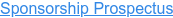# CDAO New Zealand

3 - 5 November 2020
New Zealand's premier event for the data and analytics industry
GOING ONLINE FOR 2020true true true true true true true true true true true true true true true true true true true true true true true true true true true true true true true true true true true true true true true true true true true true true true true true true true true true true true true true true true true true true true true true true true true true true true true true true true true true true true true true true true true true true true true true true true true true true true true true true true